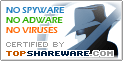# CALCULATOR Pro

SNAPSHOT

Scientific Calculator that solves math. terms

Windows AllPlatform :
\$0Price :
4.98 MBFile Size :
ScreenshotScreenshot :Popularity :Calculator Pro is a calculator tool for windows like a common pocket calculator. Calculator Pro gives you the possibility to calculate substantial mathematical terms in an easy and efficient way. Therefore Calculator Pro supports a lot of mathematical functions like trigonometric, hyperbolic functions etc. The advantage of the Calculator Pro compared with the other calculators like the Standard Windows Calculator for example, is that you can see the term you want to solve and you can combine almost an arbitrary amount of single functions. To keep that term clearly arranged the Calculator Pro support brackets. An addition, the Calculator Pro contains a list of more than 50 mathematical and scientific constants. These constants can be handled in the term like numbers and can be entered direct form a popup menu.

• InterReg
Interpolation and Regression Curve Fitting
• AcaStat Mac
Inexpensive and easy-to-use statistical tool. Academic pricing. Mac and Windows.
Propagates a laser beam from source to target
• Math-Tac-Toe Desktop
Math-Tac-Toe Desktop
• CalcExp
A Powerful Java Science Calculator.
• TQ's FunPlot
It is a function plotting software that plots any user-typed 1-D and 2-D functions. The generated plots are fully-interactive and document-ready.
• Rent Calculator Plus!
Rent Calculator Plus! Let us do the math.
• 3DMath Explorer
3DMath Explorer 3D Graph Plotting Software
• Quick Calculus
The simplest software for solving calculus.
• Calc Pilot
Calc Pilot allows you to perform calculations inside a document.